Posted 26 Aug 2018 by Nikolay with 6 Comments  |  Updated 20 Mar 2019

The indirect addressing can be used to save time and effort or simplify and reduce your source code.

If you have a PLC with 40 Analog Inputs you can use the indirect addressing to process the information coming from the Analog Inputs, like scaling, checking of alarm limit values etc., so for example you don’t have to program the alarm limits evaluation for each signal separately.

In a few steps I will show how to do a simple indirect addressing.

Demo 1

### Indirect addressing done in Studio 5000

First in the Tags Editor window we have to define one variable of type array, which in this example has twenty elements ARRAY. For your application you can define array of any type, even of user defined type (UDT).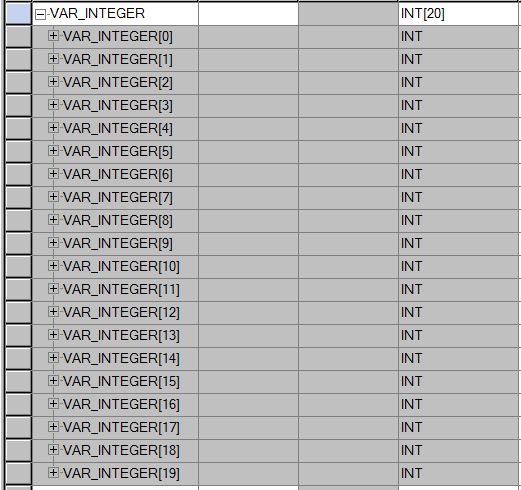Figure 1. Array of integers in Studio5000.

Sot let’s assume that during the run of my program, event triggered, I want to put information in the array, and each time in the next element.

I am going to declare one additional variable “Index” of type integer.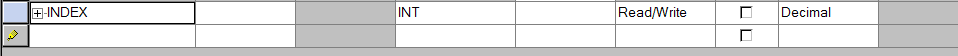Figure 2. Index variable in Studio5000.

This variable is going to be used like “Place holder” for the actual number of the ARRAY elements - VAR_INTEGER[INDEX]. If the value of variable INDEX is 10, when reading from or writing to VAR_INTEGER[INDEX], we actually  manipulate element number 10 of the array VAR_INTEGER.

In the following piece of code, you see that when the variable TRIGGER is activated the value Machine_VALUE is moved once int the corresponding array element and the INDEX variable is incremented by one.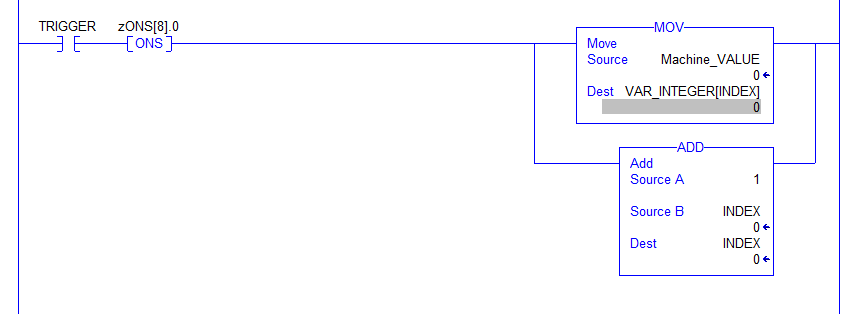Figure 3. Writing machine data in the array elements.

In the next step we are going to initialize the INDEX after reaching the maximum number of the array elements.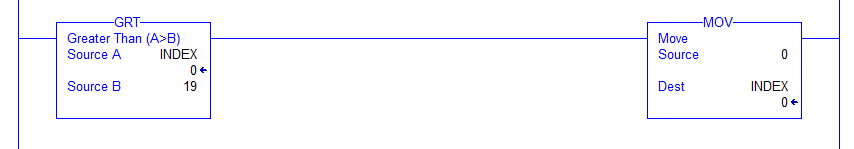Figure 4. Index initialization in Studio5000.

Demo 2

### Indirect addressing done in SIEMENS TIA PORTAL

In the second demo is identical to the first one, just done in TIA portal, it also applies to the S7 1500 and S7 1200 Controllers as “Optimized block access” is used. First I generated some PLC variables which are used to control the loop.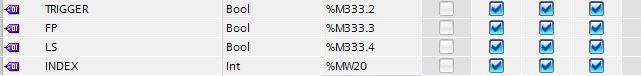Figure 5. PLC variables in TIA Portal.

After that I generated a Data Block (DB) – DB_Memory and in the DB Array variable (VAR_INTEGER) of type Integer. I this example I have used 20 elements.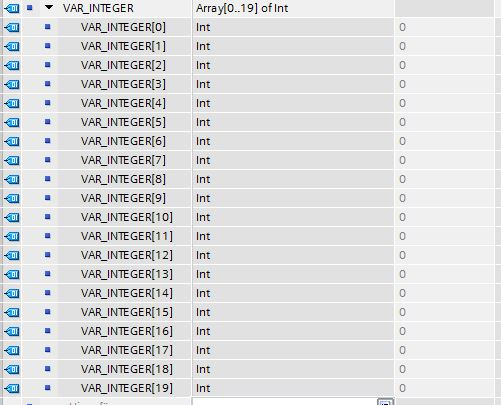Figure 6. Array of Integer.

The variable INDEX (of type Integer) is used to address the single elements in the Array variable VAR_INTEGER.

In the data block DB_Memory I placed the variable Machine_VALUE, which is going to be written in the array elements.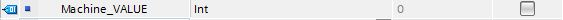Figure 7. Simulated  machine data.

In the final step I have programmed a simple loop. When the variable TRIGGER is activated the Machine_VALUE is going to be moved to the array element corresponding to the value of the variable INDEX. If the value of INDEX is 5 then the Machine_VALUE is going to be moved in array element VAR_INTEGER .

I am using ADD function to increment the INDEX variable by one. Add compare function to initialise the INDEX variable after reaching the number of elements in the array variable. In my array there are 20 variables (from 0 to 19), so if the INDEX variable is greater than 19, it receives the value “zero” and the loop is going to be reinitialized.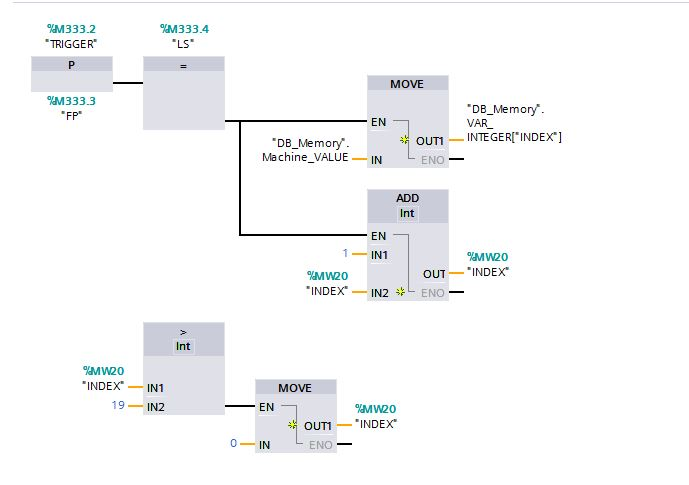Figure 8. Write data in array elements. TIA Portal.

In order to use this function with S7 300 or S7 400 PLC you have to program the “Indexing” in SCL (another way to do it is by using indirect addressing with pointer, but I wouldn’t recommend using pointer with S7 1500 & S7 1200 PLCs as it impacts the CPU’s performance).

First I generate a Function block using SCL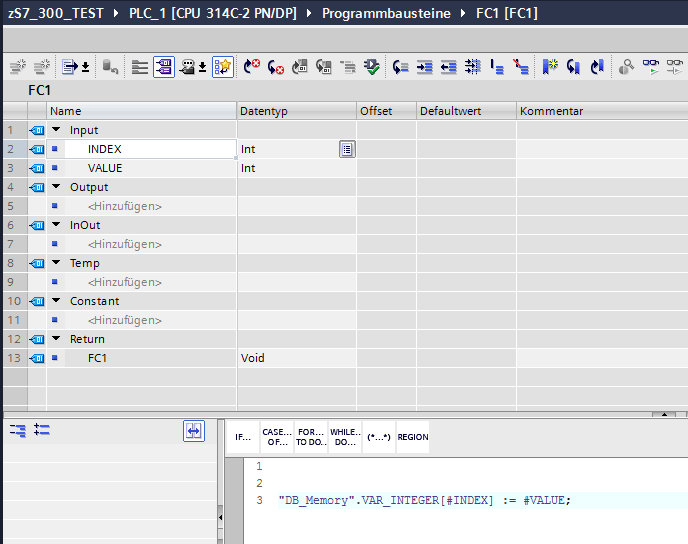Figure 9. FC1 indirect addressing, passing value to the indexed element.

FC1 has two inputs “INDEX” and “VALUE”. INDEX is used to replace the number of the ARRAY elements: If INDEX = 5, then we are writing to the array element VAR_INTEGER.
VALUE
is the integer value to be written in the array elements.

The you call this function in your program code. It does not matter if you are programming in function block, ladder or structured text for example.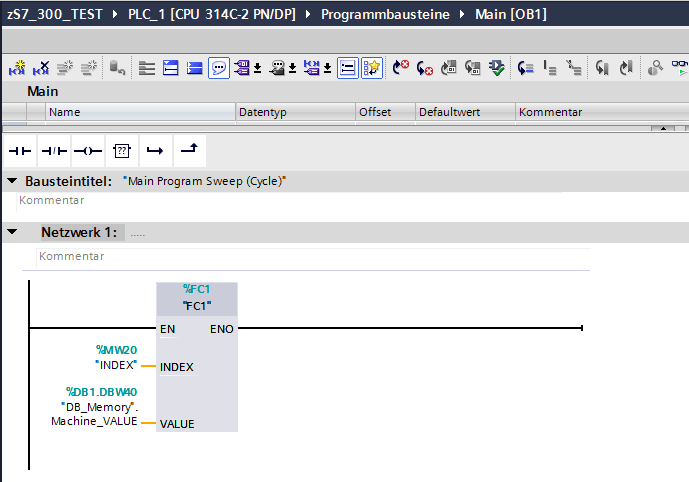Figure 10. Calling FC1 in the program code.

Compared to the previous example, here is used FC1 instead of the MOVE function. The rest of the program remains the same.

This function could be used in S7 classic. First we need the DB and the INDEX variable.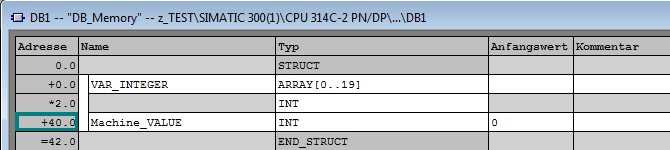Figure 11. Variable DB.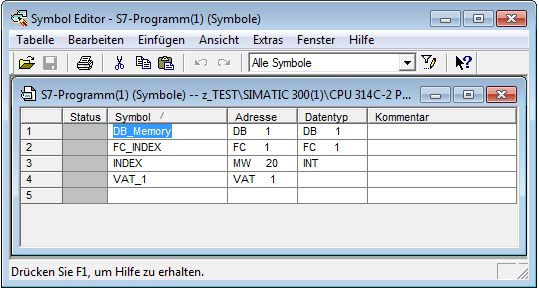Figure 12. Index variable.

Than I program the simple function in SCL.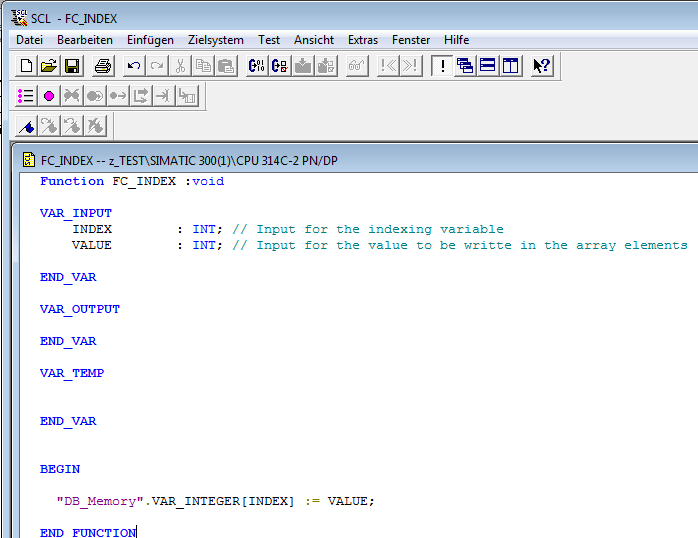Figure 13. FC1 indirect addressing, passing value to the indexed element in S7-classic.

Just like in the previous example, we call this function in the program code.

The two examples show how to make a simple loop, which for example can be used as shift register to store machine data in array variable.

### Join the discussion

vijay  14 Mar 2019

`It is not working for S7-300. Plz help...I want in LAD form`

Nikolay Bozov 20 Mar 2019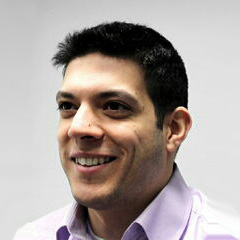Hello vijay ,to be able to pragram this function in S7 300 you have to do it in SCL. I have updated the article to show how it could be done.Regards

Nursultan  31 Jul 2019

`Hello Mr. Bozov,I've got one problem while working with this array; the softwaredoesn't accept the tag "DB"Array[index] thing. Asks for a particularnumber. What do you think, what is the problem? And have you tried thesame mechanism with array inputs? For example, several same pumps andjust one function with only index changing.Best regards.`

Nikolay Bozov 11 Aug 2019Hello Nursultan,Yes you can use this function to control several pumps. Basically this is the idea, if you for example have 10 pumps, you can define Array of type PUMP (where PUMP is UDT - User Defined Type) and then use index variable to  point to the specified Pump.Could you please give some more information so I can help you with the software. Are programming in TIA or in S7 Classic? Also which programming language are you using?Regards

vishakha jagtap  05 Jan 2022

If i want to write same logic in ladder , in simatic manager, how to
do that?

Nikolay Bozov 05 Jan 2022Hello vishakha jagtap, You can do it as shown in the example. It does not matter in which Programming language you are working.  Just program the funciton block as shown and then in Ladder use Move funciton to increase the index.Regards, Nikolay
Latest posts //

See article

See article

#### Earthing and Grounding systems

See article

Education is an admirable thing, but it is well to remember from time to time that nothing that is worth knowing can be taught.

- Oscar Wilde

Did you know?

At its centre the Sun reaches temperatures of 15 million °C and it is essentially all colors mixed together, which appear to our eyes as white.